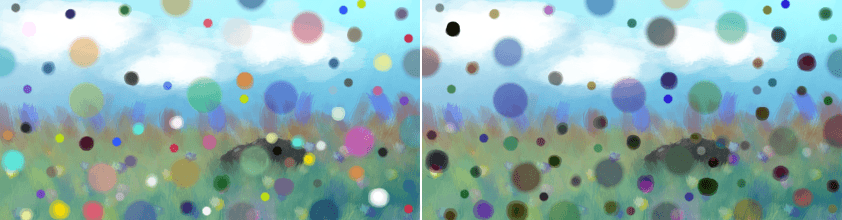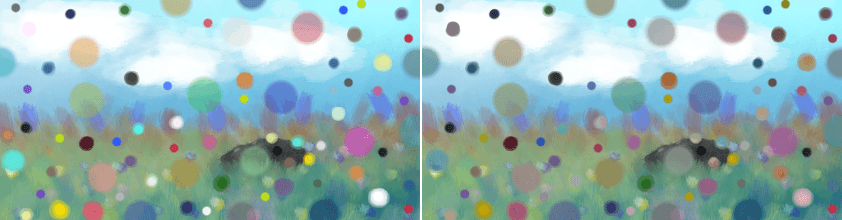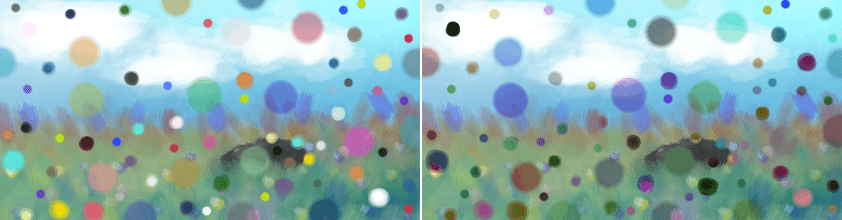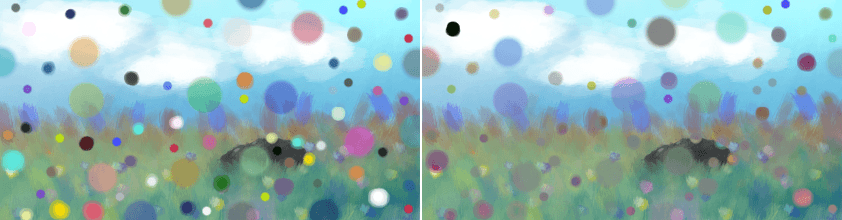# Negative¶

These are all blending modes which seem to make the image go negative.

## Additive Subtractive¶

Subtract the square root of the lower layer from the upper layer.Left: Normal. Right: Additive Subtractive.

## Arcus Tangent¶

Divides the lower layer by the top. Then divides this by Pi. Then uses that in an Arc tangent function, and multiplies it by two.Left: Normal. Right: Arcus Tangent.

## Difference¶

Checks per pixel of which layer the pixel-value is highest/lowest, and then subtracts the lower value from the higher-value.Left: Normal. Right: Difference.

## Equivalence¶

Subtracts the underlying layer from the upper-layer. Then inverts that. Seems to produce the same result as Difference.Left: Normal. Right: Equivalence.

## Exclusion¶

This multiplies the two layers, adds the source, and then subtracts the multiple of two layers twice.Left: Normal. Right: Exclusion.

## Negation¶

The absolute of the 1.0f value subtracted by base subtracted by the blend layer. abs(1.0f - Base - Blend)Left: Normal. Right: Negation.